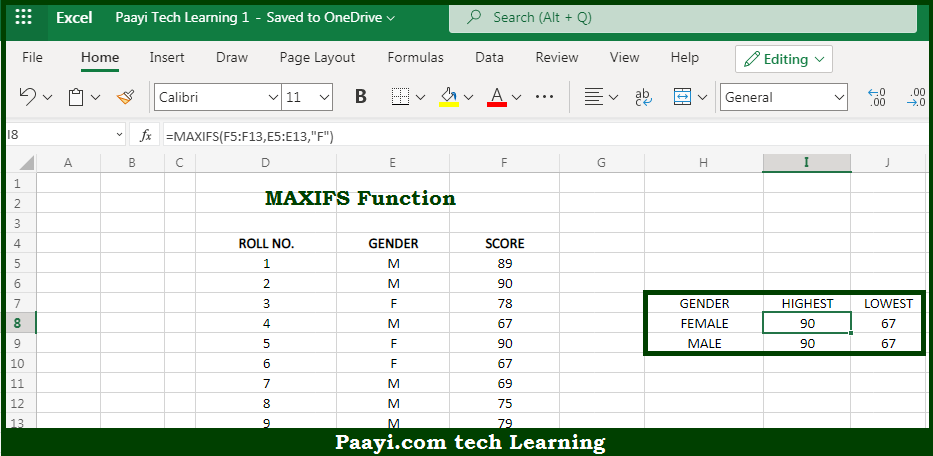# Learn How to Use Microsoft Excel MAXIFS Function

Written by | 0 Comments | 536 Views

In this article, you will learn how to use the Microsoft Excel MAXIFS function and its prime function in Microsoft Excel. You will also get to know the Microsoft Excel MAXIFS function return value and syntax with the help of some examples.

Microsoft Excel MAXIFS Function

The main purpose of the Microsoft Excel MAXIFS function is to get the maximum value with the criteria. That implies, with the help of the MAXIFS function you can able to return the largest numeric value that meets one or more criteria in a range of values provided. It should be noted that the MAXIFS function can also be used with criteria based on dates, numbers, text, and other conditions. The MAXIFS function supports logical operators (>,<,<>,=) and wildcards (*,?) for partial matching. So, with the help of the MAXIFS function, you can able to get the largest value with the criteria.

Return Value of MAXIFS Function

The return value will be the largest value.

Syntax of MAXIFS Function

=MAXIFS(max-range, range1, criteria1, [range2], [criteria2], ...)

Where the arguments:

• max-range: This is the range or value used to determine the maximum.
• range1: This is the first range to evaluate.
• criteria1: This is the criteria to use on range1.
• range2: This is the second range to evaluate.
• criteria2: This is the criteria to use on range2.

How to Use Microsoft Excel MAXIFS Function?So we know that Microsoft Excel MAXIFS function you can able to get the maximum value with the criteria. That implies, with the help of the MAXIFS function you can able to return the largest numeric value that meets one or more criteria in a range of values provided. It should be noted that the MAXIFS function can also be used with criteria based on dates, numbers, text, and other conditions. The MAXIFS function supports logical operators (>,<,<>,=) and wildcards (*,?) for partial matching. So, with the help of the MAXIFS function, you can able to get the largest value with the criteria.